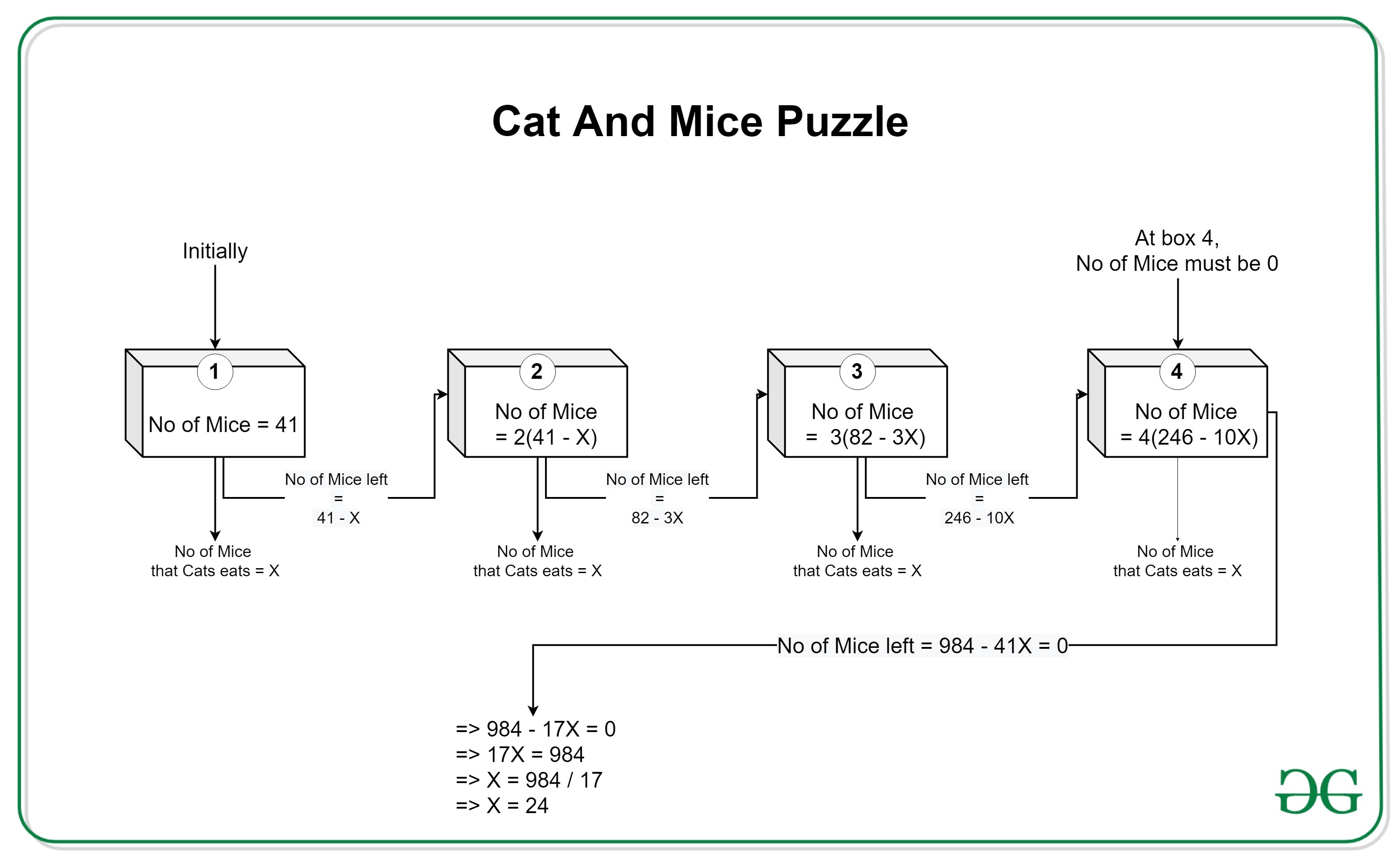# Puzzle | Cat, Mice and 4 Boxes

Puzzle:

There are 4 boxes numbered 1, 2, 3, and 4. Initially, there are 41 mice inside Box 1. A Cat comes and eats X number of mice from Box 1 and destroys it. The remaining mice from Box 1 go into Box 2 and multiply 2 times. The Cat returns in a few days and eats X mice again, destroying the Box 2. Again the leftover mice will go into Box 3 and multiply 3 times. The terror of Cat hits again. She returns and eats X mice again, and the process repeats. The mice go into Box 4 and multiply 4 times. But this time, when the Cat returns and eats X mice, there are no mice left in the box.

We have to find out X, i.e. the number of mice the Cat eats each time.

Solution:• Box 1:

=> Number of mice in the box initially = 41 (given)
=> Number of mice Cat eats = X (given)
=> Therefore, Number of mice left = 41 – X

• Box 2:

=> Number of mice in the box initially = 2(41 – X) = 82 – 2X
=> Number of mice Cat eats = X (given)
=> Therefore, Number of mice left = 82 – 2XX = 82 – 3X

• Box 3:

=> Number of mice in the box initially = 3(82 – 3X) = 246 – 9X
=> Number of mice Cat eats = X (given)
=> Therefore, Number of mice left = 246 – 9XX = 246 – 10X

• Box 4:

=> Number of mice in the box initially = 4(246 – 10X) = 984 – 40X
=> Number of mice Cat eats = X (given)
=> Therefore, Number of mice left = 984 – 40XX = 984 – 41X

• Result:
=> Since, given that there is no mice left in the Box 4 after Cat eats X mice from it.
=> Therefore, Number of mice left = 0
=> But from last equation, it can be seen that,
Number of mice left = 984 – 41X
=> Therefore, 984 – 41X = 0
=> Upon solving, X = 24

Therefore, the Cat eats 24 mice each time.

My Personal Notes arrow_drop_upCheck out this Author's contributed articles.

If you like GeeksforGeeks and would like to contribute, you can also write an article using contribute.geeksforgeeks.org or mail your article to contribute@geeksforgeeks.org. See your article appearing on the GeeksforGeeks main page and help other Geeks.

Please Improve this article if you find anything incorrect by clicking on the "Improve Article" button below.

Article Tags :
Practice Tags :

3

Please write to us at contribute@geeksforgeeks.org to report any issue with the above content.